﻿ MechDesigner Reference & User Interface > Dialog-Boxes > Dialog: Function-Block: Polynomial-Fit

# Dialog: Function-Block: Polynomial-Fit

Navigation:  MechDesigner Reference & User Interface > Dialog-Boxes >

# Dialog: Function-Block: Polynomial-Fit

## Polynomial-Fit FBA Polynomial Fit FB fits a series of Polynomials (5th order) to data at its input-connector. You can: •Transfer the Polynomials directly to MotionDesigner as a new Motion.•Save the motion-values at its output-connector as a text-file.•Save the motion-derivatives at the beginning and end of each Segment as a CSV file•Save the motion-derivatives at the beginning and end of each segment in the format required for a Schneider-Electric® EPAS4 servo-controller. STEP 1: Connect a wire to the input-connector of the Polynomial-Fit FB from the output-connector of a different FB.STEP 2: Open the Polynomial-Fit dialog-box: (See)

### Polynomial-Fit dialog-boxThe Polynomial-Fit dialog-box: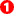Toolbar - acquire and save results.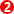Result Analysis - RMS errors and the number of Polynomials.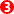Settings - tolerances, limits, and range for polynomial fitting.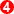Graph Plots: for the input-data and the calculated data from the Polynomials.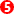Toolbar - view and save the graph-data in different ways.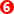Graph Legend.

#### The SettingParameters to enter before you click Fit Polynomials to Data (buttonin the Polynomial Fit toolbar, below)Position, Velocity, Acceleration buttons. Click each button to define to which motion-derivatives you want to fit polynomials.Position, Velocity, Acceleration Tolerance % Enter the tolerance, as %, for each motion-derivative, within which the Polynomials should represent the data at the input-connector.Machine Angle Range Reduce the range for which you want to calculate Polynomials - Where is the Start-Angle?Number of Points The number of data points that are calculated from the Polynomials, to plot the graphs.Point in Tolerance Sample of Points The number of points that the algorithm uses to find each polynomial to the data at the input-connector.Maximum number of Polynomials. Limits the number of Polynomials you want to fit to the data..

#### Acquire and Save toolbarAcquire and Save Results toolbar The toolbar buttons are:Acquire (Capture, Get One Cycle) button : Click this button to acquire data at the input-connector for one machine-cycle - this is the Raw-Data.Fit Polynomials to Data button: Click each time you want to Fit Polynomials to the Raw-Data.Stop Algorithms (see also Auto-Run) the algorithms will usually fit polynomials withing a few seconds. If you do not see the results, then click this button to stop the algorithms.Save Coefficients : Click to save the results to a CSV file, or a EPAS4 file that is suitable for a Schneider Electric servo-controller.Auto-Run: Immediately allow the algorithms to find polynomials fit the data to the accuracy as specified, and with less or equal to the maximum number of polynomials.Send Polynomials to MotionDesigner : Add a new Motion name-tab, and send the Polynomials as a new motion.

#### Result AnalysisRMS Position Error, RMS Velocity Error, RMS Acceleration Error (Read-Only). They give the RMS (Root, Mean, Square) error values of the Position, Velocity and Acceleration between the 'best-polynomial-fit' and the 'input-motion-data', as percentage of the maximum range of each motion-derivative. Number of Fitted Polynomials in Fit The number of Polynomials in the numerical solution. See also: Maximum Polynomial Count. in the Settings for Polynomial Fitting Algorithms.

#### Acquire and Save toolbarThe toolbar buttons are:Print Graphs :  Print the graphs immediately to the default printerSave Graph Data: Save the Data to a text file. each time you want to Fit Polynomials to the Raw-Data.Zoom Out and Zoom In to the Graph DetailsPan Left and Pan Right : Click to save the results to a CSV file, or a EPAS4 file that is suitable for a Schneider Electric servo-controller.Zoom ExtentsDisplay Graphs as: Toggle between Plot Above each other and Plot Stacked on-top of each other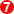Show or Hide Graph Legend.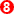X-Y Data at active cursor. Click on one of the three graphs, to activate a cursor. Then move yo